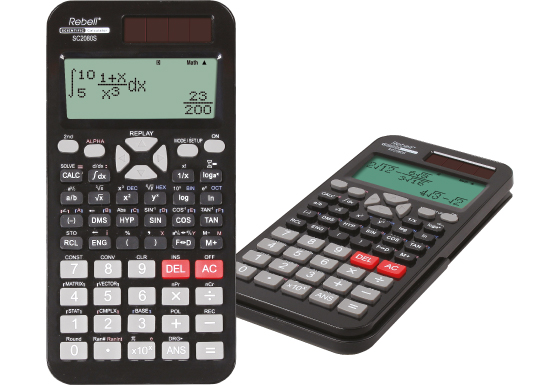## SC 2080S

Advanced scientific calculator SC 2080S performs 417 built-in functions including system of linear equations, matrix and vector calculations, numeric derivative and integral, metric conversions and physical constants. Large dot matrix display makes it possible to write and display expressions as on paper, dual power saves the backup battery and sturdy plastic cover protects the calculator against scratches and falls.Specification

• Built-In Functions: 417
• Entry System: Algebraic (D.A.L.)
• Power Source: Solar & Battery
• Dimensions and Weight: 162 × 82 × 18 mm, 129 g

Mathematical Functions

• Fractions
• Function Table
• Equation Solver (Newton)
• System of Linear Equations
• Matrix and Vector Calculations
• Numeric Derivative and Integral
• Complex Number Calculation

Statistics

• 1-Variable Statistics
• 2-Variable Statistics
• Regression Calculations
• Mean, Standard Deviation
• nCr, nPr, n!
• Random Numbers

Number Systems

• Number Systems: DEC, BIN, OCT, HEX, PEN
• Logical Operation (AND, OR, NOT, NEG, XOR)

Memory

• Multi-Line Playback
• Memory Variables: 9

Notation and Decimal Places

• FSE Display (SCI, FIX, ENG)

Trigonometric Functions